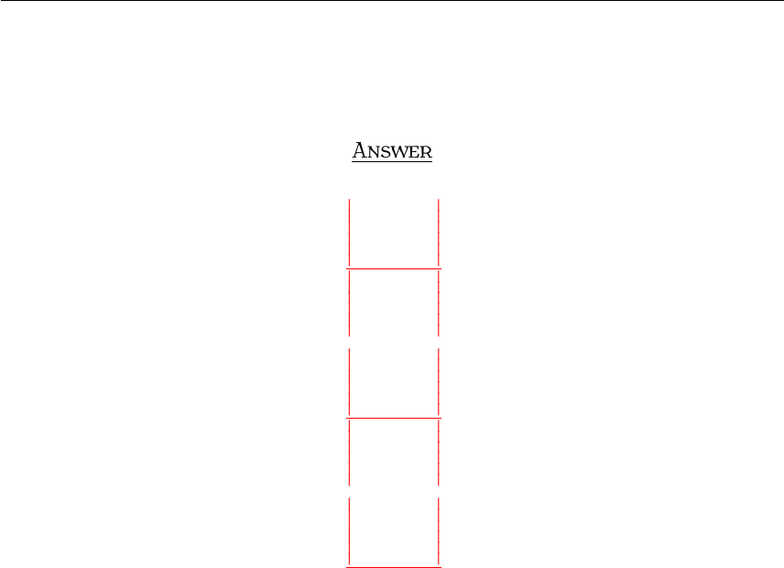Study Guides (400,000)
US (230,000)
BC (3,000)
MATH (90)
All (80)
Midterm

# Exam3210Sample4AnsExam

Department
Mathematics
Course Code
MATH 2211
Professor
All
Study Guide
Midterm

This preview shows pages 1-2. to view the full 6 pages of the document.MT T  S 
I S. Y
A , 
S, N:
Q . THE PROPERTIES OF DETERMINANTS
An n×nmatrix A is called skew-symmetric if At=A. Show that if Ais skew-symmetric and nis an
odd positive integer, then Ais not invertible.
A
By the properties of determinant, det(At) = det(A)Ñdet(A) = det(A)Ñdet(A) = (1)ndet(A)Ñ
det(A) = det(A). So, we get det(A) = 0 which implies that Ais not invertible. Note that Ameans that
EVERY ROW of Ais multiplied by -1.
1

Only pages 1-2 are available for preview. Some parts have been intentionally blurred.Q . CRAMER’S RULE, VOLUME, AND LINEAR TRANSFORMATIONS
Solve the linear system using Cramer’s Rule:
x1+ 2x2+ 3x3= 6
2x2+ 3x3= 5
x3= 1
A
x1=
6 2 3
5 2 3
1 0 1
1 2 3
0 2 3
0 0 1
= 1,
x2=
1 6 3
0 5 3
0 1 1
1 2 3
0 2 3
0 0 1
= 1,
x3=
1 2 6
0 2 5
0 0 1
1 2 3
0 2 3
0 0 1
= 1.
Therefore, (1,1,1) is the solution of the given system.
2